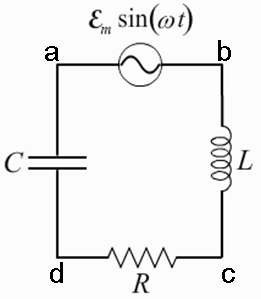# Problem: A circuit is constructed with an AC generator, a resistor, capacitor and inductor as shown. The generator voltage varies in time as ε =Va - Vb = εmsinωt, where εm = 120 V and ω = 737 radians/second. The values for the remaining circuit components are: R = 83 Ω, L = 112.5 mH, and C = 11.6μF.Which of the following statements is true?a)The voltage across the generator is zero when the magnitudes of the voltages across the inductor and the capacitor are maximum.b)The current in the circuit is zero when the magnitudes of the voltages across the inductor and the capacitor are maximum.c)The magnitude of the voltage across the generator is maximum when the magnitudes of the voltages across the inductor and the capacitor are maximum.d)The magnitude of the current in the circuit is maximum when the magnitudes of the voltages across the inductor and the capacitor are maximum.e)There is no time when the magnitudes of the voltages across the inductor and capacitor are maximum.

###### FREE Expert Solution

Phase angle:

$\begin{array}{rcl}{\mathbf{\varphi }}& \mathbf{=}& {{\mathbf{tan}}}^{\mathbf{-}\mathbf{1}}\frac{{\mathbf{X}}_{\mathbf{L}}\mathbf{-}{\mathbf{X}}_{\mathbf{C}}}{\mathbf{R}}\\ & \mathbf{=}& {{\mathbf{tan}}}^{\mathbf{-}\mathbf{1}}\frac{{\mathbf{\left(}}{\mathbf{737}}{\mathbf{×}}{\mathbf{112}}{\mathbf{.}}{\mathbf{5}}{\mathbf{×}}{{\mathbf{10}}}^{\mathbf{-}\mathbf{3}}{\mathbf{-}}\frac{\mathbf{1}}{\mathbf{737}\mathbf{×}\mathbf{11}\mathbf{.}\mathbf{6}\mathbf{×}{\mathbf{10}}^{\mathbf{-}\mathbf{6}}}{\mathbf{\right)}}}{\mathbf{83}}\end{array}$

φ = -22.3°

99% (152 ratings)###### Problem Details

A circuit is constructed with an AC generator, a resistor, capacitor and inductor as shown. The generator voltage varies in time as ε =Va - Vb = εmsinωt, where εm = 120 V and ω = 737 radians/second. The values for the remaining circuit components are: R = 83 Ω, L = 112.5 mH, and C = 11.6μF.Which of the following statements is true?

a)The voltage across the generator is zero when the magnitudes of the voltages across the inductor and the capacitor are maximum.

b)The current in the circuit is zero when the magnitudes of the voltages across the inductor and the capacitor are maximum.

c)The magnitude of the voltage across the generator is maximum when the magnitudes of the voltages across the inductor and the capacitor are maximum.

d)The magnitude of the current in the circuit is maximum when the magnitudes of the voltages across the inductor and the capacitor are maximum.

e)There is no time when the magnitudes of the voltages across the inductor and capacitor are maximum.

Frequently Asked Questions

What scientific concept do you need to know in order to solve this problem?

Our tutors have indicated that to solve this problem you will need to apply the Series LRC Circuits concept. You can view video lessons to learn Series LRC Circuits. Or if you need more Series LRC Circuits practice, you can also practice Series LRC Circuits practice problems.